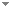Switch to: References

# Index sets for Π01 classes

Annals of Pure and Applied Logic 93 (1-3):3-61 (1998)

 Ordinal Analysis of Partial Combinatory Algebras.Paul Shafer & Sebastiaan A. Terwijn - 2021 - Journal of Symbolic Logic 86 (3):1154-1188.For every partial combinatory algebra, we define a hierarchy of extensionality relations using ordinals. We investigate the closure ordinals of pca’s, i.e., the smallest ordinals where these relations become equal. We show that the closure ordinal of Kleene’s first model is ${\omega _1^{\textit {CK}}}$ and that the closure ordinal of Kleene’s second model is $\omega _1$. We calculate the exact complexities of the extensionality relations in Kleene’s first model, showing that they exhaust the hyperarithmetical hierarchy. We also discuss embeddings of (...) Direct download (2 more)     Export citationBookmarkDensity of the Medvedev Lattice of Π0 1 Classes.Douglas Cenzer & Peter G. Hinman - 2003 - Archive for Mathematical Logic 42 (6):583-600.The partial ordering of Medvedev reducibility restricted to the family of Π0 1 classes is shown to be dense. For two disjoint computably enumerable sets, the class of separating sets is an important example of a Π0 1 class, which we call a c.e. separating class''. We show that there are no non-trivial meets for c.e. separating classes, but that the density theorem holds in the sublattice generated by the c.e. separating classes. Direct download (3 more)     Export citationBookmark17 citations The Complexity of Recursive Constraint Satisfaction Problems.Victor W. Marek & Jeffrey B. Remmel - 2010 - Annals of Pure and Applied Logic 161 (3):447-457.We investigate the complexity of finding solutions to infinite recursive constraint satisfaction problems. We show that, in general, the problem of finding a solution to an infinite recursive constraint satisfaction problem is equivalent to the problem of finding an infinite path through a recursive tree. We also identify natural classes of infinite recursive constraint satisfaction problems where the problem of finding a solution to the infinite recursive constraint satisfaction problem is equivalent to the problem of finding an infinite path through (...) Direct download (5 more)     Export citationBookmarkPAC Learning, VC Dimension, and the Arithmetic Hierarchy.Wesley Calvert - 2015 - Archive for Mathematical Logic 54 (7-8):871-883.We compute that the index set of PAC-learnable concept classes is m-complete Σ30\documentclass[12pt]{minimal} \usepackage{amsmath} \usepackage{wasysym} \usepackage{amsfonts} \usepackage{amssymb} \usepackage{amsbsy} \usepackage{mathrsfs} \usepackage{upgreek} \setlength{\oddsidemargin}{-69pt} \begin{document}$${\Sigma^{0}_{3}}$$\end{document} within the set of indices for all concept classes of a reasonable form. All concept classes considered are computable enumerations of computable Π10\documentclass[12pt]{minimal} \usepackage{amsmath} \usepackage{wasysym} \usepackage{amsfonts} \usepackage{amssymb} \usepackage{amsbsy} \usepackage{mathrsfs} \usepackage{upgreek} \setlength{\oddsidemargin}{-69pt} \begin{document}$${\Pi^{0}_{1}}$$\end{document} classes, in a sense made precise here. This family of concept classes is sufficient to cover all standard examples, and also has the property that PAC learnability (...) Direct download (7 more)     Export citationBookmarkEffectively Closed Sets and Enumerations.Paul Brodhead & Douglas Cenzer - 2008 - Archive for Mathematical Logic 46 (7-8):565-582.An effectively closed set, or ${\Pi^{0}_{1}}$ class, may viewed as the set of infinite paths through a computable tree. A numbering, or enumeration, is a map from ω onto a countable collection of objects. One numbering is reducible to another if equality holds after the second is composed with a computable function. Many commonly used numberings of ${\Pi^{0}_{1}}$ classes are shown to be mutually reducible via a computable permutation. Computable injective numberings are given for the family of ${\Pi^{0}_{1}}$ classes and (...) Direct download (4 more)     Export citationBookmark1 citation